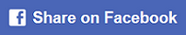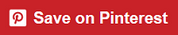﻿ 2 digit addition without / with regrouping games ONLINE

Page No. 1002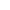------------------------------------------
New game

Drag the digits on the left into the correct boxes.

## 2 digit addition games ONLINE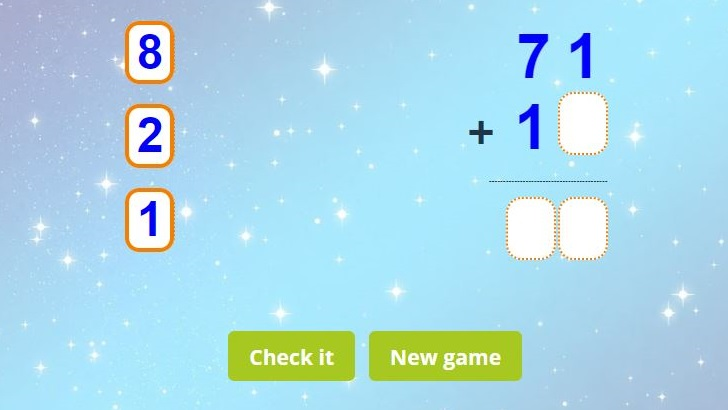Learn 2 digit addition with and without regrouping: use the digits on the left side to complete the two digit addition problem on the right side. Drag each digit into the correct position. Submit your answer and check the result.

• ### 2 digit by 2 digit addition without regrouping games

Click on the blue button No regrouping and play two digit addition without regrouping games. 2 digit addition without regrouping is when the digits add up to a number that is 9 or less. The sum can be written below each place value column. Add within 100 without regrouping are online math games for 2nd graders.

• ### 2 digit addition with regrouping games

Click on the blue button Regrouping and you can play two digit addition with regrouping games online. 2 digit addition with regrouping means that the sum of the digits in one column is more than 9, we carry forward the number to the left column: regrouping forward occurs from the ones column to the tens column.

## Printable 2 digit addition worksheets PDF

I have included in these 2 digit by 2 digit addition worksheets both with regrouping and without regrouping pages. So you can start off simple and increase the challenge as your students are ready.

Generate free printable two digit addition worksheets in PDF below. These double digit addition worksheets help you to practice two digit addition in the classroom or at home. These two digit addition worksheets contain also the answer key. You can find more addition worksheets here.

## 2 digit addition without regrouping ONLINE games

Two digit addition games are the first step for mastering column addition. When kids are familiar with this method they can easily continue to 3 digit addition games.

• Start by moving the first number from the left column into the addition problem.
• Check if the result can be formed from the 2 remaining numbers of the left column.
• If not, return the number to its original position and repeat the procedure with the second number.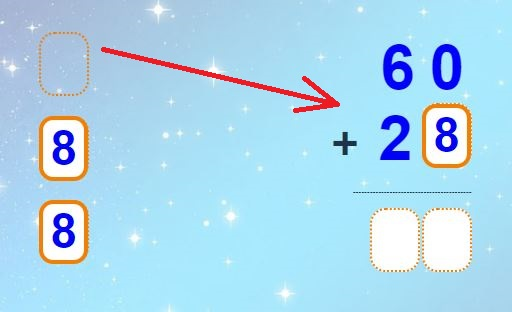## Adding 2 digit numbers with regrouping

Addition of 2 digit numbers can be played online on any device or it can be practiced in the classroom with the help of this 2 digit addition game. These two digit addition games will make learning of 2 digit addition much more interesting.

How to play two digit addition games in the classroom?

You will need:

### Play double digit addition games ONLINE

1. Generate the new 2 digit addition problem. Simply press the button NEW GAME.
2. Place 3 numbers (displayed in the left column) on the magnetic board.
3. Use a whiteboard pen to write down the two digit addition task and draw the empty boxes.
4. Call on 1 student to solve the double digit addition problem.
5. The student have to place the 3 magnetic numbers into the empty boxes so that the result of the addition of 2 digit numbers is correct: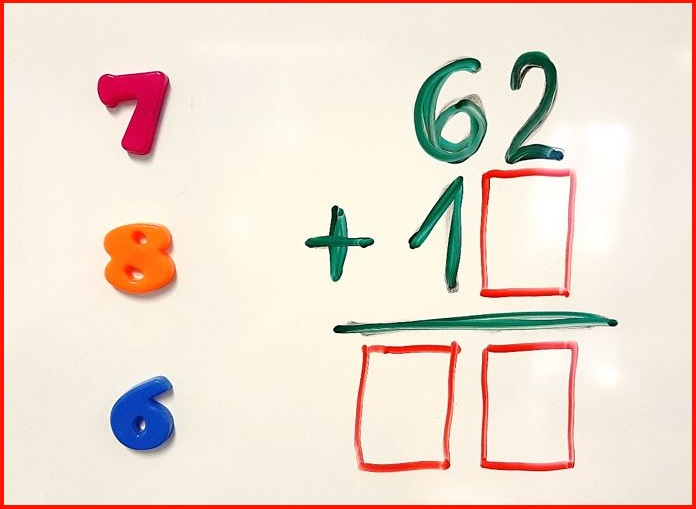Two digit addition games can be played also "offline". 🙂

## 2 digit addition online games

For practicing two digit addition within 100 with or without regrouping you can play also these online games: addition pyramids puzzles, numbers bonds to 100 games or try these online addition flash cards up to 100.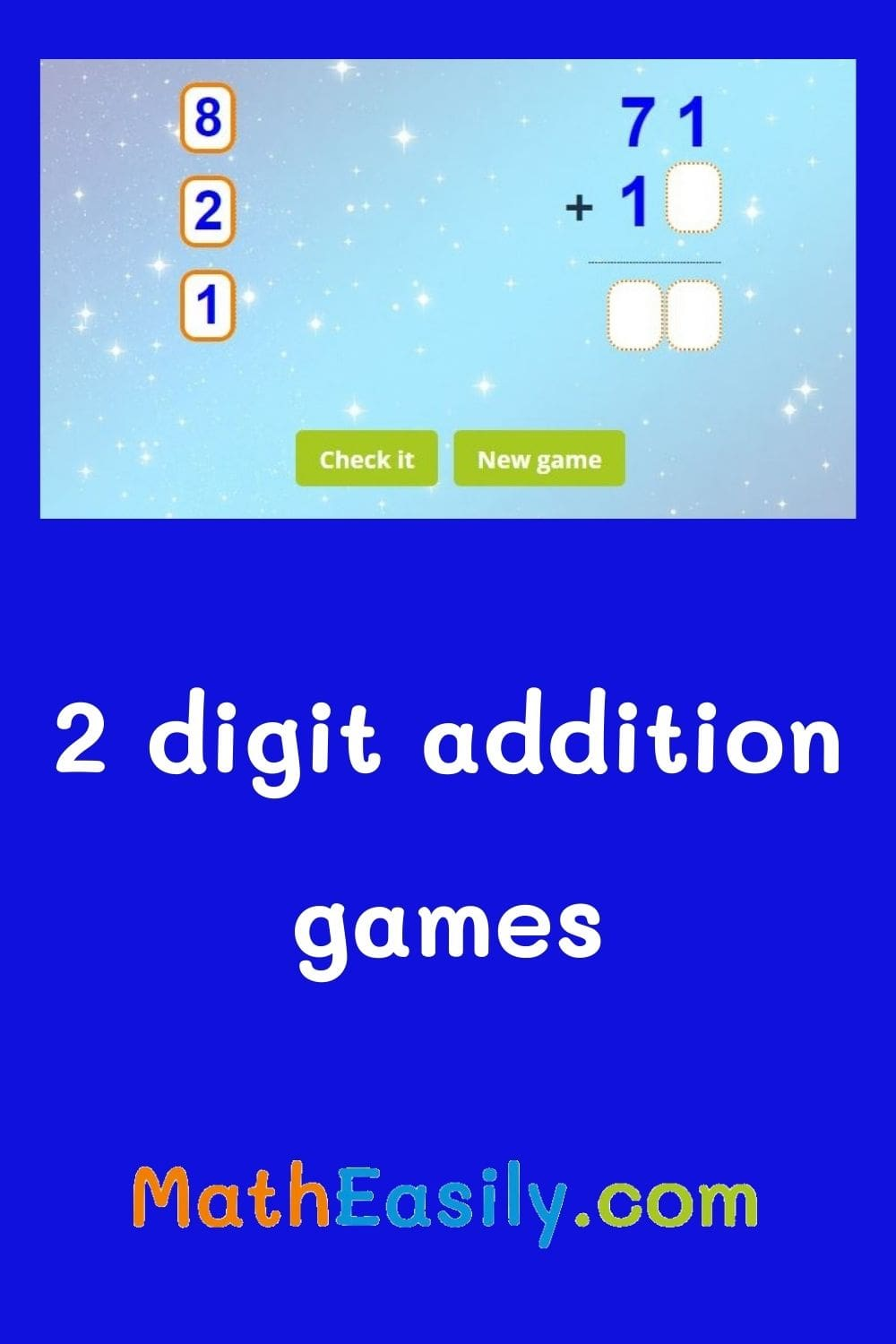## 2 digit addition WITH regrouping

Our two digit addition online games develop students' logic as well as memory.

Children learn to look for a solution that will lead them to the right result. As in everyday life.

### What is 2 digit addition?

Two-digit addition is a basic arithmetic operation in mathematics that involves adding two numbers that have two digits each.

For example, if you have the numbers "23" and "45", you would add them together to find the sum of "68". To do this, you line up the digits in each number so that the "ones" place is lined up with the "ones" place and the "tens" place is lined up with the "tens" place.

Then, you start by adding the ones place together and carrying over any extra amount to the next place value if needed.

The process continues until you have added all the digits together and found the final sum.

Two-digit addition is an important foundation for more advanced arithmetic operations and helps children learn the basics of carrying and borrowing in arithmetic.

## Adding two digit numbers games

This online addition game involves solving 2 digit addition problems by dragging and dropping the correct answer into a designated box.